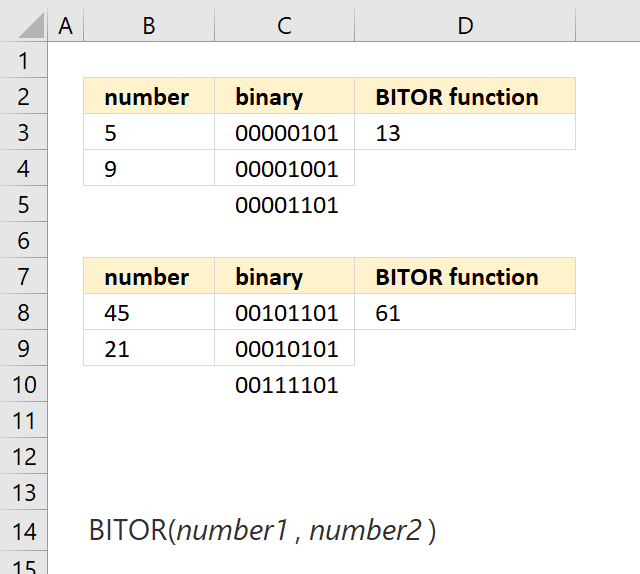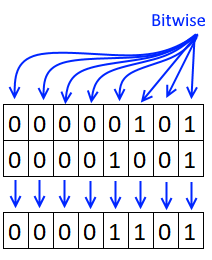Author: Oscar Cronquist Article last updated on May 11, 2022The BITOR function performs a bitwise 'OR' of two numbers.

Bitwise meaning each digit in the binary equivalent.

Formula in cell D3:

=BITOR(B3, B4)

Number 5 is 101 binary and number 9 is 1001. If at least one bit is 1 the returning digit is 1.101 + 1101 = 1101. 1101 is number 13.

### Excel Function Syntax

BITOR(number1, number2)

### Arguments

 number1 Required. The first number. number1 Required. The second number.